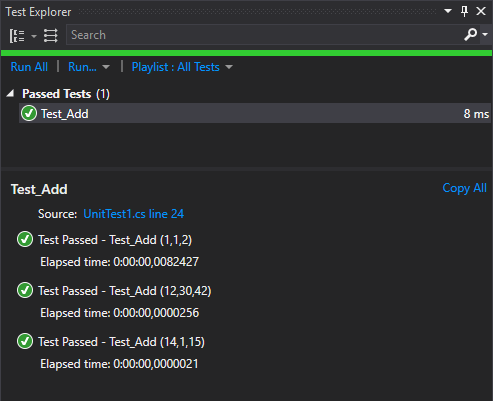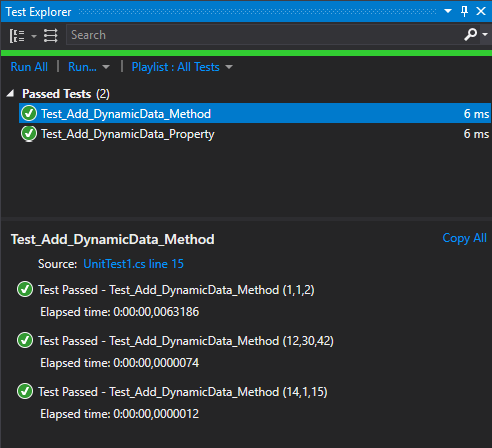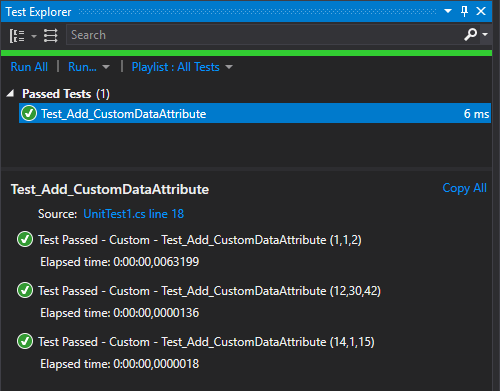# MSTest v2: Data tests

• Gérald Barré

This post is part of the series 'MSTest v2'. Be sure to check out the rest of the blog posts of the series!

In the previous post, I showed you the basis of writing a unit test with MSTest v2. There was only one unit test for the `MathHelper.Add` method. Of course, you want to write more tests to validate the behavior of the method. One way is to copy and paste the method and change the value of the parameters.

C#
``````[TestClass]
public class MathTests
{
[TestMethod]
{
var actual = MathHelper.Add(1, 1);
var expected = 2;
Assert.AreEqual(expected, actual);
}

[TestMethod]
{
var actual = MathHelper.Add(21, 4);
var expected = 25;
Assert.AreEqual(expected, actual);
}
}``````

As a developer, you may avoid copy-pasting code every time it's possible! This idea is to create a parameterized test. This means the test methods have parameters and uses them to parametrize the test. This way, one test method can be used to run N tests. MSTest v2 provides 3 ways to create parametrized tests.

## #Using DataRow

The `[DataRow]` attribute allows setting the values of the parameter of the test. You can set as many `[DataRow]` attributes as you want. Also, you need to replace `[TestMethod]` with `[DataTestMethod]`:

C#
``````[TestClass]
public class MathTests
{
[DataTestMethod]
[DataRow(1, 1, 2)]
[DataRow(12, 30, 42)]
[DataRow(14, 1, 15)]
public void Test_Add(int a, int b, int expected)
{
var actual = MathHelper.Add(a, b);
Assert.AreEqual(expected, actual);
}
}``````

You can see that there are 3 tests in the test explorer.Note that MS Tests supports `params` parameters, so you can use it to define easily arrays:

C#
``````[TestClass]
public class MathTests
{
[DataTestMethod]
[DataRow(1, new [] { "a", "b" })] // Explicit array construction
[DataRow(1, "a", "b")]            // It will create the array automatically
public void Test_Add(int a, params string[] b)
{
// TODO actual test
}
}``````

## #Using DynamicData

If your data cannot be set into an attribute parameter (non-constant values or complex objects), you can use the `[DynamicData]` attribute. This attribute allows getting the values of the parameters from a method or a property. The method or the property must return an `IEnumerable<object[]>`. Each row corresponds to the values of a test.

Here's an example with a method:

C#
``````[TestClass]
public class MathTests
{
[DataTestMethod]
[DynamicData(nameof(GetData), DynamicDataSourceType.Method)]
public void Test_Add_DynamicData_Method(int a, int b, int expected)
{
var actual = MathHelper.Add(a, b);
Assert.AreEqual(expected, actual);
}

public static IEnumerable<object[]> GetData()
{
yield return new object[] { 1, 1, 2 };
yield return new object[] { 12, 30, 42 };
yield return new object[] { 14, 1, 15 };
}
}``````

Here's an example with a property:

C#
``````[TestClass]
public class MathTests
{
[DataTestMethod]
[DynamicData(nameof(Data), DynamicDataSourceType.Property)]
public void Test_Add_DynamicData_Property(int a, int b, int expected)
{
var actual = MathHelper.Add(a, b);
Assert.AreEqual(expected, actual);
}

public static IEnumerable<object[]> Data
{
get
{
yield return new object[] { 1, 1, 2 };
yield return new object[] { 12, 30, 42 };
yield return new object[] { 14, 1, 15 };
}
}
}``````## #Custom DataSource

If the 2 previous attributes don't fit your needs, you can create your attribute. The attribute must implement `ITestDataSource`. This interface has 2 methods: `GetData` and `GetDisplayName`. `GetData` returns the data rows. `GetDisplayName` returns the name of the test for a data row. This name is visible in the Test Explorer or the console.

C#
``````public class CustomDataSourceAttribute : Attribute, ITestDataSource
{
public IEnumerable<object[]> GetData(MethodInfo methodInfo)
{
yield return new object[] { 1, 1, 2 };
yield return new object[] { 12, 30, 42 };
yield return new object[] { 14, 1, 15 };
}

public string GetDisplayName(MethodInfo methodInfo, object[] data)
{
if (data != null)
return string.Format(CultureInfo.CurrentCulture, "Custom - {0} ({1})", methodInfo.Name, string.Join(",", data));

return null;
}
}``````

Then, you can use this attribute as any other data attributes:

C#
``````[DataTestMethod]
[CustomDataSource]
public void Test_Add(int a, int b, int expected)
{
var actual = MathHelper.Add(a, b);
Assert.AreEqual(expected, actual);
}``````## #Conclusion

You can easily create a lot of unit tests using parametrized tests. The 2 attributes `DataRow` and `DynamicData` should be enough for most of the cases. The data tests are extensible, so you can create your attributes to create your data source. Of course, you can combine the 3 methods for the same test method.

Do you have a question or a suggestion about this post? Contact me!